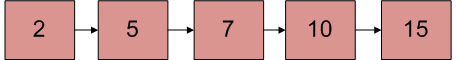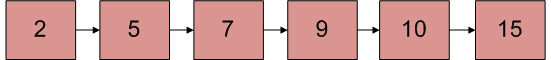# Given a linked list which is sorted, how will you insert in sorted way

Given a sorted linked list and a value to insert, write a function to insert the value in a sorted way.Linked List after insertion of 9## Recommended: Please solve it on “PRACTICE” first, before moving on to the solution.

Algorithm:
Let input linked list is sorted in increasing order.

```1) If Linked list is empty then make the node as
2) If the value of the node to be inserted is smaller
than the value of the head node, then insert the node
at the start and make it head.
3) In a loop, find the appropriate node after
which the input node (let 9) is to be inserted.
To find the appropriate node start from the head,
keep moving until you reach a node GN (10 in
the below diagram) who's value is greater than
the input node. The node just before GN is the
appropriate node (7).
4) Insert the node (9) after the appropriate node
(7) found in step 3.
```

Implementation:

## C++

 `/* Program to insert in a sorted list */` `#include ` `using` `namespace` `std; ` ` `  `/* Link list node */` `class` `Node { ` `public``: ` `    ``int` `data; ` `    ``Node* next; ` `}; ` ` `  `/* function to insert a new_node  ` `in a list. Note that this  ` `function expects a pointer to  ` `head_ref as this can modify the  ` `head of the input linked list  ` `(similar to push())*/` `void` `sortedInsert(Node** head_ref, ` `                  ``Node* new_node) ` `{ ` `    ``Node* current; ` `    ``/* Special case for the head end */` `    ``if` `(*head_ref == NULL ` `        ``|| (*head_ref)->data ` `               ``>= new_node->data) { ` `        ``new_node->next = *head_ref; ` `        ``*head_ref = new_node; ` `    ``} ` `    ``else` `{ ` `        ``/* Locate the node before the ` ` ``point of insertion */` `        ``current = *head_ref; ` `        ``while` `(current->next != NULL  ` `&& current->next->data  ` `< new_node->data) { ` `            ``current = current->next; ` `        ``} ` `        ``new_node->next = current->next; ` `        ``current->next = new_node; ` `    ``} ` `} ` ` `  `/* BELOW FUNCTIONS ARE JUST  ` `UTILITY TO TEST sortedInsert */` ` `  `/* A utility function to  ` `create a new node */` `Node* newNode(``int` `new_data) ` `{ ` `    ``/* allocate node */` `    ``Node* new_node = ``new` `Node(); ` ` `  `    ``/* put in the data */` `    ``new_node->data = new_data; ` `    ``new_node->next = NULL; ` ` `  `    ``return` `new_node; ` `} ` ` `  `/* Function to print linked list */` `void` `printList(Node* head) ` `{ ` `    ``Node* temp = head; ` `    ``while` `(temp != NULL) { ` `        ``cout << temp->data << ``" "``; ` `        ``temp = temp->next; ` `    ``} ` `} ` ` `  `/* Driver program to test count function*/` `int` `main() ` `{ ` `    ``/* Start with the empty list */` `    ``Node* head = NULL; ` `    ``Node* new_node = newNode(5); ` `    ``sortedInsert(&head, new_node); ` `    ``new_node = newNode(10); ` `    ``sortedInsert(&head, new_node); ` `    ``new_node = newNode(7); ` `    ``sortedInsert(&head, new_node); ` `    ``new_node = newNode(3); ` `    ``sortedInsert(&head, new_node); ` `    ``new_node = newNode(1); ` `    ``sortedInsert(&head, new_node); ` `    ``new_node = newNode(9); ` `    ``sortedInsert(&head, new_node); ` `    ``cout << ``"Created Linked List\n"``; ` `    ``printList(head); ` ` `  `    ``return` `0; ` `} ` `// This is code is contributed by rathbhupendra `

## C

 `/* Program to insert in a sorted list */` `#include ` `#include ` ` `  `/* Link list node */` `struct` `Node { ` `    ``int` `data; ` `    ``struct` `Node* next; ` `}; ` ` `  `/* function to insert a new_node ` ` ``in a list. Note that this ` `  ``function expects a pointer  ` `to head_ref as this can modify the ` `  ``head of the input linked  ` `list (similar to push())*/` `void` `sortedInsert(``struct` `Node** head_ref, ` `                  ``struct` `Node* new_node) ` `{ ` `    ``struct` `Node* current; ` `    ``/* Special case for the head end */` `    ``if` `(*head_ref == NULL ` `        ``|| (*head_ref)->data ` `               ``>= new_node->data) { ` `        ``new_node->next = *head_ref; ` `        ``*head_ref = new_node; ` `    ``} ` `    ``else` `{ ` `        ``/* Locate the node before  ` `the point of insertion */` `        ``current = *head_ref; ` `        ``while` `(current->next != NULL ` `               ``&& current->next->data < new_node->data) { ` `            ``current = current->next; ` `        ``} ` `        ``new_node->next = current->next; ` `        ``current->next = new_node; ` `    ``} ` `} ` ` `  `/* BELOW FUNCTIONS ARE JUST UTILITY TO TEST sortedInsert */` ` `  `/* A utility function to create a new node */` `struct` `Node* newNode(``int` `new_data) ` `{ ` `    ``/* allocate node */` `    ``struct` `Node* new_node  ` `= (``struct` `Node*)``malloc``( ` `sizeof``(``struct` `Node)); ` ` `  `    ``/* put in the data  */` `    ``new_node->data = new_data; ` `    ``new_node->next = NULL; ` ` `  `    ``return` `new_node; ` `} ` ` `  `/* Function to print linked list */` `void` `printList(``struct` `Node* head) ` `{ ` `    ``struct` `Node* temp = head; ` `    ``while` `(temp != NULL) { ` `        ``printf``(``"%d  "``, temp->data); ` `        ``temp = temp->next; ` `    ``} ` `} ` ` `  `/* Driver program to test count function*/` `int` `main() ` `{ ` `    ``/* Start with the empty list */` `    ``struct` `Node* head = NULL; ` `    ``struct` `Node* new_node = newNode(5); ` `    ``sortedInsert(&head, new_node); ` `    ``new_node = newNode(10); ` `    ``sortedInsert(&head, new_node); ` `    ``new_node = newNode(7); ` `    ``sortedInsert(&head, new_node); ` `    ``new_node = newNode(3); ` `    ``sortedInsert(&head, new_node); ` `    ``new_node = newNode(1); ` `    ``sortedInsert(&head, new_node); ` `    ``new_node = newNode(9); ` `    ``sortedInsert(&head, new_node); ` `    ``printf``(``"\n Created Linked List\n"``); ` `    ``printList(head); ` ` `  `    ``return` `0; ` `} `

## Java

 `// Java Program to insert in a sorted list ` `class` `LinkedList { ` `    ``Node head; ``// head of list ` ` `  `    ``/* Linked list Node*/` `    ``class` `Node { ` `        ``int` `data; ` `        ``Node next; ` `        ``Node(``int` `d) ` `        ``{ ` `            ``data = d; ` `            ``next = ``null``; ` `        ``} ` `    ``} ` ` `  `    ``/* function to insert a  ` `new_node in a list. */` `    ``void` `sortedInsert(Node new_node) ` `    ``{ ` `        ``Node current; ` ` `  `        ``/* Special case for head node */` `        ``if` `(head == ``null` `|| head.data  ` `>= new_node.data) { ` `            ``new_node.next = head; ` `            ``head = new_node; ` `        ``} ` `        ``else` `{ ` ` `  `            ``/* Locate the node before point of insertion. */` `            ``current = head; ` ` `  `            ``while` `(current.next != ``null`  `&& current.next.data < new_node.data) ` `                ``current = current.next; ` ` `  `            ``new_node.next = current.next; ` `            ``current.next = new_node; ` `        ``} ` `    ``} ` ` `  `    ``/*Utility functions*/` ` `  `    ``/* Function to create a node */` `    ``Node newNode(``int` `data) ` `    ``{ ` `        ``Node x = ``new` `Node(data); ` `        ``return` `x; ` `    ``} ` ` `  `    ``/* Function to print linked list */` `    ``void` `printList() ` `    ``{ ` `        ``Node temp = head; ` `        ``while` `(temp != ``null``) { ` `            ``System.out.print(temp.data + ``" "``); ` `            ``temp = temp.next; ` `        ``} ` `    ``} ` ` `  `    ``/* Driver function to test above methods */` `    ``public` `static` `void` `main(String args[]) ` `    ``{ ` `        ``LinkedList llist = ``new` `LinkedList(); ` `        ``Node new_node; ` `        ``new_node = llist.newNode(``5``); ` `        ``llist.sortedInsert(new_node); ` `        ``new_node = llist.newNode(``10``); ` `        ``llist.sortedInsert(new_node); ` `        ``new_node = llist.newNode(``7``); ` `        ``llist.sortedInsert(new_node); ` `        ``new_node = llist.newNode(``3``); ` `        ``llist.sortedInsert(new_node); ` `        ``new_node = llist.newNode(``1``); ` `        ``llist.sortedInsert(new_node); ` `        ``new_node = llist.newNode(``9``); ` `        ``llist.sortedInsert(new_node); ` `        ``System.out.println(``"Created Linked List"``); ` `        ``llist.printList(); ` `    ``} ` `} ` `/* This code is contributed by Rajat Mishra */`

## Python

 `# Python program to insert in a sorted list ` ` `  `# Node class  ` `class` `Node: ` ` `  `    ``# Constructor to initialize the node object ` `    ``def` `__init__(``self``, data): ` `        ``self``.data ``=` `data ` `        ``self``.``next` `=` `None` ` `  `class` `LinkedList: ` ` `  `    ``# Function to initialize head ` `    ``def` `__init__(``self``): ` `        ``self``.head ``=` `None` ` `  `    ``def` `sortedInsert(``self``, new_node): ` `         `  `        ``# Special case for the empty linked list  ` `        ``if` `self``.head ``is` `None``: ` `            ``new_node.``next` `=` `self``.head ` `            ``self``.head ``=` `new_node ` ` `  `        ``# Special case for head at end ` `        ``elif` `self``.head.data >``=` `new_node.data: ` `            ``new_node.``next` `=` `self``.head ` `            ``self``.head ``=` `new_node ` ` `  `        ``else` `: ` ` `  `            ``# Locate the node before the point of insertion ` `            ``current ``=` `self``.head ` `            ``while``(current.``next` `is` `not` `None` `and` `                 ``current.``next``.data < new_node.data): ` `                ``current ``=` `current.``next` `             `  `            ``new_node.``next` `=` `current.``next` `            ``current.``next` `=` `new_node ` ` `  `    ``# Function to insert a new node at the beginning ` `    ``def` `push(``self``, new_data): ` `        ``new_node ``=` `Node(new_data) ` `        ``new_node.``next` `=` `self``.head ` `        ``self``.head ``=` `new_node ` ` `  `    ``# Utility function to print it the linked LinkedList ` `    ``def` `printList(``self``): ` `        ``temp ``=` `self``.head ` `        ``while``(temp): ` `            ``print` `temp.data, ` `            ``temp ``=` `temp.``next` ` `  ` `  `# Driver program ` `llist ``=` `LinkedList() ` `new_node ``=` `Node(``5``) ` `llist.sortedInsert(new_node) ` `new_node ``=` `Node(``10``) ` `llist.sortedInsert(new_node) ` `new_node ``=` `Node(``7``) ` `llist.sortedInsert(new_node) ` `new_node ``=` `Node(``3``) ` `llist.sortedInsert(new_node) ` `new_node ``=` `Node(``1``) ` `llist.sortedInsert(new_node) ` `new_node ``=` `Node(``9``) ` `llist.sortedInsert(new_node) ` `print` `"Create Linked List"` `llist.printList() ` ` `  `# This code is contributed by Nikhil Kumar Singh(nickzuck_007) `

## C#

 `// C# Program to insert in a sorted list ` `using` `System; ` ` `  `public` `class` `LinkedList { ` `    ``Node head; ``// head of list ` ` `  `    ``/* Linked list Node*/` `    ``class` `Node { ` `        ``public` `int` `data; ` `        ``public` `Node next; ` `        ``public` `Node(``int` `d) ` `        ``{ ` `            ``data = d; ` `            ``next = ``null``; ` `        ``} ` `    ``} ` ` `  `    ``/* function to insert a new_node in a list. */` `    ``void` `sortedInsert(Node new_node) ` `    ``{ ` `        ``Node current; ` ` `  `        ``/* Special case for head node */` `        ``if` `(head == ``null` `|| head.data >= new_node.data) { ` `            ``new_node.next = head; ` `            ``head = new_node; ` `        ``} ` `        ``else` `{ ` ` `  `            ``/* Locate the node before  ` `            ``point of insertion. */` `            ``current = head; ` ` `  `            ``while` `(current.next != ``null` `&& current.next.data < new_node.data) ` `                ``current = current.next; ` ` `  `            ``new_node.next = current.next; ` `            ``current.next = new_node; ` `        ``} ` `    ``} ` ` `  `    ``/*Utility functions*/` ` `  `    ``/* Function to create a node */` `    ``Node newNode(``int` `data) ` `    ``{ ` `        ``Node x = ``new` `Node(data); ` `        ``return` `x; ` `    ``} ` ` `  `    ``/* Function to print linked list */` `    ``void` `printList() ` `    ``{ ` `        ``Node temp = head; ` `        ``while` `(temp != ``null``) { ` `            ``Console.Write(temp.data + ``" "``); ` `            ``temp = temp.next; ` `        ``} ` `    ``} ` ` `  `    ``/* Driver code */` `    ``public` `static` `void` `Main(String[] args) ` `    ``{ ` `        ``LinkedList llist = ``new` `LinkedList(); ` `        ``Node new_node; ` ` `  `        ``new_node = llist.newNode(5); ` `        ``llist.sortedInsert(new_node); ` ` `  `        ``new_node = llist.newNode(10); ` `        ``llist.sortedInsert(new_node); ` ` `  `        ``new_node = llist.newNode(7); ` `        ``llist.sortedInsert(new_node); ` ` `  `        ``new_node = llist.newNode(3); ` `        ``llist.sortedInsert(new_node); ` ` `  `        ``new_node = llist.newNode(1); ` `        ``llist.sortedInsert(new_node); ` ` `  `        ``new_node = llist.newNode(9); ` `        ``llist.sortedInsert(new_node); ` ` `  `        ``Console.WriteLine(``"Created Linked List"``); ` `        ``llist.printList(); ` `    ``} ` `} ` ` `  `/* This code is contributed by 29AjayKumar */`

Output:

```Created Linked List
1 3 5 7 9 10 ```

Complexity Analysis:

• Time Complexity: O(n).
Only one traversal of the list is needed.
• Auxiliary Space: O(1).
No extra space is needed.

Shorter Implementation using double pointers:
Thanks to Murat M Ozturk for providing this solution. Please see Murat M Ozturk’s comment below for complete function. The code uses double-pointer to keep track of the next pointer of the previous node (after which new node is being inserted).

Note that below line in code changes current to have address of next pointer in a node.

```   current = &((*current)->next);
```

```    /* Copies the value-at-address current to
new_node's next pointer*/
new_node->next = *current;

/* Fix next pointer of the node (using its address)
after which new_node is being inserted */
*current = new_node;
```

Time Complexity: O(n)

Attention reader! Don’t stop learning now. Get hold of all the important DSA concepts with the DSA Self Paced Course at a student-friendly price and become industry ready.

My Personal Notes arrow_drop_up

Article Tags :

18

Please write to us at contribute@geeksforgeeks.org to report any issue with the above content.